# Online Algebra 2 & Trigonometry

## Get 1 on 1 help from Algebra 2 & Trigonometry tutors

Algebra 2 & Trigonometry is a necessary step in solving many real-world problems. This coursework builds upon the foundations laid out in Pre-Algebra and Algebra 1. As your student learns Algebra 2 & Trigonometry, they will cover a range of different topics, from understanding matrices to learning exponential and logarithmic functions.

They will take the concepts they learned in Pre-Algebra and Algebra 1 and build upon them. Learning Algebra 2 will help teach your student to understand equations and solve for variables. In addition to other functions, such as sequences and series, your student will also learn how to read and understand graphing functions, such as exponential graphs.

As they progress through this mathematics course, they will learn a deeper understanding of different numerical properties, preparing them for further coursework in math. When your student learns Algebra 2, they will also learn some properties of Trigonometry.

### Algebra 2 & Trigonometry

Algebra 2 & Trigonometry is a necessary step in solving many real-world problems. This coursework builds upon the foundations laid out in Pre-Algebra and Algebra 1. As your student learns Algebra 2 & Trigonometry, they will cover a range of different topics, from understanding matrices to learning exponential and logarithmic functions. They will take the concepts they learned in Pre-Algebra and Algebra 1 and build upon them.

Learning Algebra 2 will help teach your student to understand equations and solve for variables. In addition to other functions, such as sequences and series, your student will also learn how to read and understand graphing functions, such as exponential graphs. As they progress through this mathematics course, they will learn a deeper understanding of different numerical properties, preparing them for further coursework in math.

When your student learns Algebra 2, they will also learn some properties of Trigonometry. Applying knowledge about determining right angles and calculating the area of a sphere has real world applications in certain settings, such as architecture and teaching.
Through our study program with one-on-one tutoring, your student will get easy to understand guidance on topics in Algebra 2. Between studying essential concepts and working on practice problems, your student will learn all that they need to know about Algebra 2 before they move on to coursework such as Pre-Calculus.

Algebra 2 & Trigonometry are essential to understand before moving on to other mathematics courses. Your student needs a solid foundation in this coursework before moving on to more abstract courses like Calculus. Algebra 2 is generally a high school subject within countries such as the United States. Your student will go from basic understandings of linear equations to more comprehensive knowledge of topics such as quadratic equations. Once they have this coursework solidified through the help of our one-on-one tutors, they are ready to move on to other subjects, such as Trigonometry.

### Learn Algebra 2 & Trigonometry with us

Our one-on-one tutoring will help your student get a firm handle on the increasingly complex coursework that comes along with being in Algebra 2. By working in an environment with a single student, our tutors are better able to focus on providing knowledge for the student’s needs as compared to being in a classroom full of other students. Our one-on-one instruction will give your student the basics that they need to succeed in Algebra 2. We lay out all of the information that your student will need with a syllabus that provides guidance from the start.

Our tutors will help your student understand Algebra 2 concepts, no matter whether they are easy or complex. Through our one-on-one tutoring system, we walk your student through concepts that they need to grasp and work with them to solve problems. Once your student has learned the basics and reviewed problems, our tutors will assist them as necessary to review and explain any concepts as they solve problems.

With our Algebra 2 & Trigonometry tutors, your student will have someone available to assist them every step of the way, ensuring that trouble spots get addressed quickly rather than waiting until several chapters later to be discovered. Our tutors can provide problem-solving strategies with each topic so that your student understands how to apply the knowledge that they have just learned. With our problems to solve, your student can check their work to ensure they know how to get the correct answer each time, giving them greater confidence in their Algebra 2 problem-solving abilities.

Our tutoring program goes along well with any Algebra 2 program of study. As your student learns topics in the classroom, our tutors can help them shore up their skills and learn to apply concepts later that day. When they work on solving problems, our tutors can be on-hand to help them apply their newfound skills to get the correct answer. Your student is then able to apply these concepts to their regular classroom assignments.Same Tutor
Every TimeTutors with
MS DegreeSame
TextbooksManaged
TutoringVery
ConvenientAffordable
\$16/hr for 8hr Monthly
Below 8hr/Month, \$20/hr
What You’ll Learn
• Linear Systems
• Logarithmic Functions
• Probability and Statistics•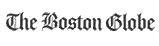•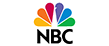••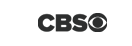•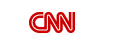•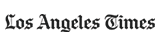•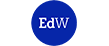•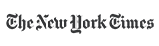•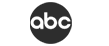### How You Benefit FromThis Program

• Flexible Scheduling
• Quality Learning
• Proven Results
• Tutors with Masters
• Affordable Price
• Dedicated Support
• Money Back Guarantee

Name*
Phone number*
Email*